Defineand compute symbolically.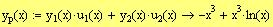Define the particular solution as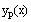and compute it symbolically.
The step below is provided to verify that the particular solution satisfies the differential equation.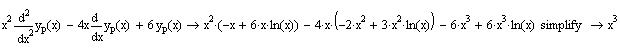_____________O_____________
3. Given that 1, -1, -1, 1+ i, 1 + i are the roots of the characteristic polynomial of a linear homogenous differential equation with real constant coefficients L y(x) = 0
a) Find the operator L using the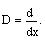notation.
Note: You may leave L in a factored form but make sure that it does not involve complex numbers.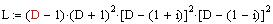Define the operator L using the given roots.
Note: The fact that the differential equation has only real coefficient means that complex roots appear in conjugates.
Use the "expand" command to display the operator L.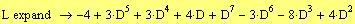Remark: L may also be defined in the following manner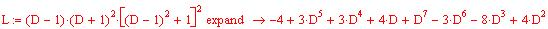b) Write the general solution of the differential equation.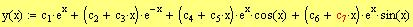_____________O_____________
4. Given the linear system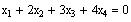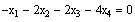a ) Find a basis for the solution space of the system
The system will be solved by the "Given .. Find ( )" command.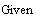Extra:
We may check if we got the right answer as follows: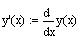Define y'(x) and y''(x) as the first and second derivative of y(x), respectively and evaluate symbolically to see the computed derivatives.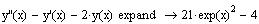Substitute in the left-hand side of the differential equation and use the "expand" command. The answer should be equal to the right-hand side of the equation.
_____________O____________
2. Given that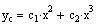is the complementary solution of the differential equation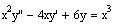find a particular solution of the equation using the method of variation of parameters.
Solution: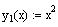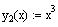Define the two solution of the associated homogeneous equation as y1(x) and y2(x).Define the nonhomogeneous term as f(x) after dividing the equation by the coefficient of the highest derivative.
Define the Wronskian matrix as W(x) and evaluate symbolically to display the result.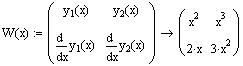Compute the Wronskian as the determinant of W(x) and evaluate symbolically.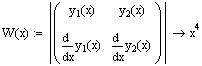Define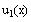and compute symbolically.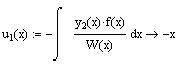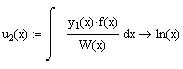Define two vectors u an v elements in the vector space V. Apply the condition on their components.and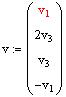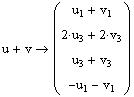Compute the sum u + v, symbolically.
The sum vector satisfies the conditions on the components of the elements of V. Therefore, V is closed under addition.
Make the decision whether V is closed under addition based on whether the components of the sum vector satisfies the conditions.
Compute a scalar (k) multiple of a vector in v and make the decision whether V is closed under scalar multiplication based on whether the components of kv satisfies the conditions.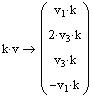The components of a scalar multiple of a vector in V
satisfies the conditions. Therefore, V is closed under
scalar multiplication.
Therefore, V is a subspace of R4 .
_____________O_____________
6. Justify without solving that the vectors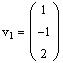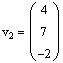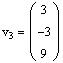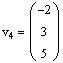are linearly dependent.
We have for vectors in R3 . Therefore, using the theorem that states: "any n + 1 vectors in Rn are linearly dependent," the vectors are linearly dependent.
_____________O_____________
7. Answer the following questions with (T)rue or (F)alse.

1. ____T____ In an n-dimensional vector space V, a vector in V can be written in one and only one way as a linear combination of a set of n linearly independent vectors in V.

2. ____F____ The dimension of a vector space V is the number of elements in V.

3. ____F____ In an n-dimensional vector space, a set of n-1 vectors must be linearly dependent.

4. ____T____ Every subspace of a vector space contains the zero vector.

5. ____T____ If a set S spans the vector space V, then S contains a bases for V.

6. ____F____ Any set of vectors that spans a vector space V must be linearly independent.

7. ____F____ Any linearly independent set of vectors in Rn forms a basis for Rn

8. ____F____ If a set S in a vector space V is linearly independent, then it contains a basis for V.

9. ____T____ The principle of superposition states that if x and y are two solutions to a homogeneous system, then any linear combination of x and y is also a solution to the system.

10. ____T____ The solution space of a homogeneous linear system of 3 equations in 4 unknowns is a subspace of R4Copy the equations given above and enclose them between the keywords Given and Find(var1 , var2,....), where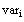stands for the variable names you are solving for. Use symbolic evaluation to find the solution.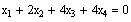Note: Another way to solve the system is to use the "solve" command as show below.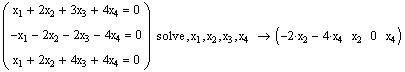To get the basis vectors, separate the solution vector along the free variables.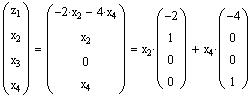Thus, the basis vectors are
Give the basis vectors.and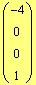b) Justify (without computation) that the basis vectors are linearly independent.
They are linearly independent because one is not a scalar multiple of the other.
c) What is the dimension of the solution space? Why?
The dimension of the solution space is 2 since the basis contains 2 vectors.
_____________O_____________
5. Let V be the set of all vectors in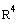such that: if v =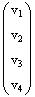e V, thenand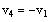. Show that V is a subspace of.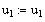This is used to reset the variable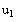since it was assigned a value before.
Let u an v be two vectors in V, thenDefine the two solutions as y1 (x) and y2 (x) in terms of the roots r1 and r2 , then use the symbolic evaluation to display the two solutions.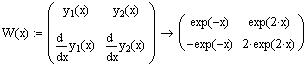Define the Wronskian matrix as W(x) and evaluate symbolically to display the results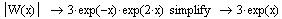Compute the determinant of W(x) to find the Wronskian of the two solutions. Use the "simplify" command to simplify the result.
Because the exponential function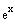is never zero, t he two solutions are linearly independent for all real values of x.
e) Give the interval over which the two solutions of the homogeneous equation are linearly independentAll real numbers since ex is never zero.
f) Use following steps to find the general solution of the nonhomogeneous equation using the method of undetermined coefficients:
f1. Write the unmodified form of the particular solution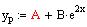f2. Write the modified form of the particular solution yp(x)
The exponential term is multiplied by x because it appears in the complementary solution due to the root "2" having multiplicity 1.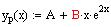f3. Compute the first and second derivative of yp(x)
Define the first derivative as y'p (x) and evaluate symbolically to display the computed derivative.Define the second derivative as the derivative of the first derivative and evaluate symbolically.Note: You may also compute y''p (x) using the second order derivative of yp(x).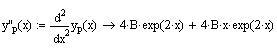Math260-041 (A. Farhat)
Major 2 Solutions
Dec. 25, 2004
Remark:
1. The exam is solved, in most part, using Mathcad. The first problem was designed and solved so that it can be used as a template for the computer project.

2. Each step of the solution is commented. Comments on steps displaying long results are placed in the middle of the page rather that to the right
1. Execute the following steps to find a solution to the differential equation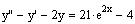satisfying the initial conditions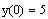a) Write the associated homogeneous equation
y'' - y' -2y = 0
b) Find the roots of the characteristic equation
Define r as the auxiliary equation and use the "solve" command to find the roots. This way the results will be assigned to r as a vector and the solutions can then be referred to using vector subscripts of r.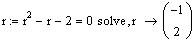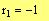Display the roots using subscripts of r.
c) Write the complementary solution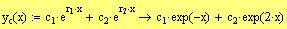Define the complementary solution asand evaluate symbolically to display the solution with the roots substituted by Mathcad.
d) Show that the two solutions of the homogeneous equation are linearly independent. Justify your answer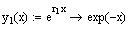Define the derivative of y(x) as y'(x) and evaluate symbolically.Evaluate the first derivative at the initial point and set it equal to the given initial value, then evaluate symbolically to get the second equation of the linear system.
g3. Write the linear system obtained in parts (g1) and (g2) as a matrix equation A c = b
This has to be done manually, but it can be automated using the "Given.. find" command.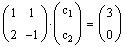g4) Form the augmented matrix of the system aboveg5) Compute the reduced row echelon form of the augmented matrix to solve the systemUse the "rref" command.
g6) Give the values of the constants c1 and c2 found above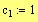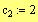Define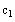and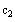based on the solution found in the previous step.
g7) Substitute the above values in the general solution of the nonhomogeneous equation to obtain the particular solutionRedefine the complementary solution as you did in part (c). This is required by Mathcad since the earlier definition of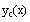was given before the c's were defined.Redefine the general solution in terms ofand(for the same reason as above).
Display the general solution. This will give the particular solution with the constants substituted in the general solution.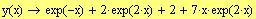f4. Substitute yp and its derivatives in the nonhomogeneous equation, simplify, and equate similar terms to determine the coefficients
Substitute yp (x) and its derivatives in the differential equation and evaluate symbolically.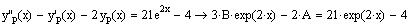To make it easy to compare similar terms, we use the command "collect" on the variable x. This will rearrange the terms as a polynomial in x. This step will help you in the computer project.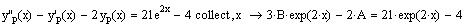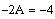Equate similar terms to find the linear system involving the undetermined coefficients. This step has to be done manually.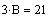Place the equations of the system in a vector and use the "solve" command to find the coefficients.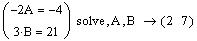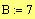Define the coefficient as solved for in the previous step.
f5. Write the particular solution after finding the coefficients
Redefine the particular solution and evaluate symbolically to display it.

Note: This redefinition is required by Mathcad so that it can substitute the values of the coefficients.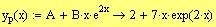f6. Write the general solution of the nonhomogeneous equation
Define the general solution y(x) as the sum of the complementary solution and the particular solution and evaluate symbolically to display the result.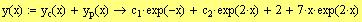g) Use the following steps to find a particular solution of the nonhomogeneous equation satisfying the given initial conditions:
g1. Apply the first initial condition on the general solution in part f6.Evaluate y(x) at the initial point and set it equal to the initial value, then evaluate symbolically to get the first equation of the linear system involving the arbitrary constants.
g2. Compute the first derivative of the general solution in part f6 and apply the second initial condition